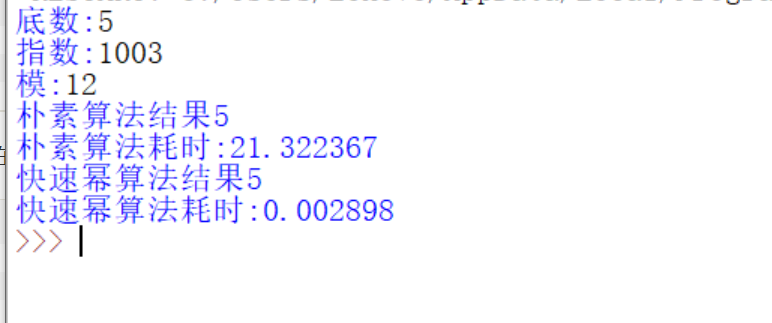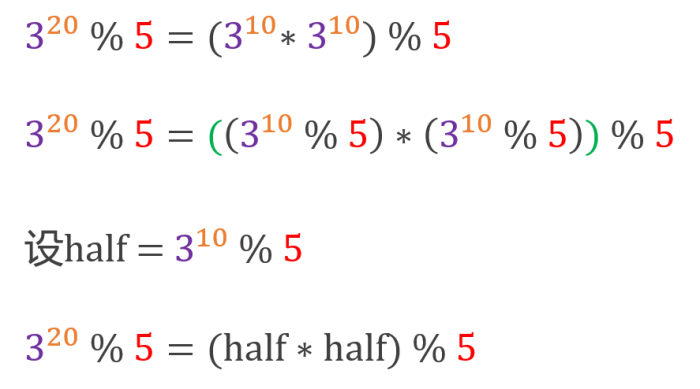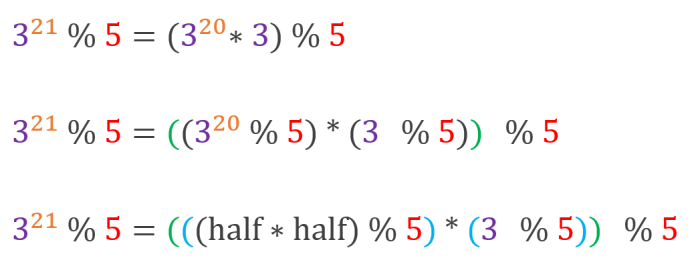• 快速幂取模算法详解
2018-08-25 16:06:00

### 快速幂取模算法详解

假设有大数a和b，无法计算a^b,那么(a^b)%c也就无法计算。例如 a = 2790,b = 2753,c = 3233

所以，有了快速幂取模算法。

#### 1，给出定理

(a^b)%c = (a%c)*(b%c)%c

#### 2,分解b

将b分解为2进制数，例如2753 = 101011000001 = 2^0 +2^6+2^7 + 2^9 +2^11

#### 3,运用公式计算

(a^b)%c = (2790* 2790^(2^6) *….)%c

= （2790%c）* (2790^(2^6)%c) *…..%c

可以看出来，即为2790的2的n次方幂 %c 一直乘的结果。

#### 4，java实现

/**
*  快速幂取模   计算 (a^b) %c
* @param a
* @param b
* @param c
* @return 计算结果
*/
private static int quick(int a,int b,int c) {
int ans=1;   //记录结果
a=a%c;   //预处理，使得a处于c的数据范围之下
while(b!=0)
{
if((b&1)==1){ //1即是0000000000000001，判断个位是否是1.如果b的二进制位是1，那么我们的结果是要参与运算的
ans=(ans*a)%c;
}
b>>=1;    //二进制的移位操作，相当于每次除以2，用二进制看，就是我们不断的遍历b的二进制位
a=(a*a)%c;   //不断的加倍
}
return ans;
}

更多相关内容
• 主要介绍了Java语言实现快速幂取模算法详解，具有一定参考价值，需要的朋友可以了解下。
• 主要介绍了C语言快速幂取模算法,包括了算法的分析与改进,是很多程序设计竞赛中常见的算法,需要的朋友可以参考下
• 我们现在知道了快速幂取模算法的强大了，我们现在来看核心原理：对于任何一个整数的模幂运算a^b%c对于b我们可以拆成二进制的形式b=b0+b1*2+b2*2^2+...+bn*2^n这里我们的b0对应的是b二进制的第一位那么我们的a^b运算...我们现在知道了快速幂取模算法的强大了，我们现在来看核心原理：

对于任何一个整数的模幂运算

a^b%c

对于b我们可以拆成二进制的形式

b=b0+b1*2+b2*2^2+...+bn*2^n

这里我们的b0对应的是b二进制的第一位

那么我们的a^b运算就可以拆解成

a^b0*a^b1*2*1...*a^(bn*2^n)

对于b来说，二进制位不是0就是1，那么对于bx为0的项我们的计算结果是1就不用考虑了，我们真正想要的其实是b的非0二进制位

那么假设除去了b的0的二进制位之后我们得到的式子是

a^(bx*2^x)*...*a(bn*2^n)

这里我们再应用我们一开始提到的公式，那么我们的a^b%c运算就可以转化为

(a^(bx*2^x)%c)*...*(a^(bn*2^n)%c)

这样的话，我们就很接近快速幂的本质了

(a^(bx*2^x)%c)*...*(a^(bn*2^n)%c)

我们会发现令

A1=(a^(bx*2^x)%c)

...

An=(a^(bn*2^n)%c)

这样的话，An始终是A(n-1)的平方倍(当然加进去了取模匀速那)，依次递推

我们可以得出以下的结论：

1.如果b是偶数，我们可以记

k = a2 mod c，那么求(k)b/2 mod c就可以了。2.如果b是奇数，我们也可以记 ((k)b/2 mod c × a ) mod   c  就可以了。

现在我们来考虑实现它：

迭代法：

1 int fast_pow(int a,int b,intc)2 {3 int ans=1; ///记录结果

4 a=a%c; ///预处理，使得a处于c的数据范围之下

5 while(b!=0)6 {7 if(b&1)///奇数

8 {9 ans=(ans*a)%c;///消除指数为奇数的影响

10 }11 b>>=1; ///二进制的移位操作,不断的遍历b的二进制位

12 a=(a*a)%c; ///不断的加倍

13 }14 returnans;15 }

递归法：

1 ll fast_pow(ll x,ll n,ll p)2 {3 ll temp;4 x=x%p;5 if(n==0)///终止条件

6 {7 return 1;8 }9 temp=fast_pow((x*x)%p,n>>1,p);10 if(n&1)11 {12 temp =temp*x%p;///消除指数为奇数的影响

13 }14 return temp%p;15 }

在这里还要进行几点说明：

1.二进制的几个运算符&  和 >> 。

&运算通常用于二进制取位操作，例如一个数 & 1 的结果就是取二进制的最末位。还可以判断奇偶，x&1==0为偶，x&1==1为奇。

>>运算比较单纯,二进制去掉最后一位，移位操作，不断遍历b的二进制位。

2. a=(a*a)%c这一步的作用是用来不断的加倍，在先不看同余定理的情况下，a*a==a^2,下一步再乘，就是a^2*a^2==a^4,然后同理  a^4 * a4 =a^8 .........?是不是做到了

a-->a^2-->a^4-->a^8-->a^16-->a^32.......指数正是 2^i 啊，再看上面的例子，a¹¹ =  a^(2^0) * a^(2^1) * a^(2^3)，这三项是不是完美解决了，快速幂就是这样。

展开全文• C++快速幂取模代码，可根据需要自行调整优化，刷题的时候计算溢出可以用它解决，算法入门代码，可以直接复制使用
• 本文是上一篇文章《程序员必学：快速幂算法》的续集，上一篇文章详细地介绍了快速幂算法，提供了递归、非递归的2种实现方案抛出问题请设计一个算法求x的y次幂模z的结果：(x ^ y) % zx、y、z都是整数z ≠ 0, y ≥ 0x...

本文是上一篇文章《程序员必学：快速幂算法》的续集，上一篇文章详细地介绍了快速幂算法，提供了递归、非递归的2种实现方案

抛出问题

请设计一个算法求x的y次幂模z的结果：(x ^ y) % z

x、y、z都是整数

z ≠ 0, y ≥ 0

x、y的绝对值可能很大，比如(1234 ^ 4567) % 30

思考

由于x、y的绝对值可能很大，x ^ y的结果可能会溢出。所以先求x ^ y，再对z取模，显然是不现实的。

这里要借助模运算的一条运算规则

(a * b) % p = ((a % p) * (b % p)) % p根据上面的推导，就可以很容易写出代码实现

递归实现

int powMod(int x, int y, int z) {

if (y == 0) return 1 % z;

int half = powMod(x, y >> 1, z);

half = (half * half) % z;

if ((y & 1) == 0) { // y是偶数

return half;

} else { // y是奇数

return (half * (x % z)) % z;

}

}

非递归实现

int powMod(int x, int y, int z) {

int result = 1 % z;

x %= z;

while (y != 0) {

if ((y & 1) == 1) {

result = (result * x) % z;

}

x = (x * x) % z;

y >>= 1;

}

return result;

}

测试用例

// 4

powMod(1234, 4567, 30);

// 699

powMod(123, 456, 789);

如果你特别希望我写点什么方面的内容，也可以留言建议，谢谢。欢迎关注

展开全文• 函数原型 power_n__module_p(x,n,p): x: 底 n: 指数 p: 模数 调用示例: power_n__module_p(3,97,353) 输出: 40
• 算法1.首先直接地来设计这个算法： int ans = 1; for(int i = 1;i<=b;i++) { ans = ans * a; } ans = ans % c; 这个算法的时间复杂度体现在for循环中，为O（b）.这个算法存在着明显的问题，如果a和b过大，...

我们先从简单的例子入手：求ab mod c = 几。

算法1.首先直接地来设计这个算法：

int ans = 1;

for(int i = 1;i<=b;i++)

{

ans = ans * a;

}

ans = ans % c;

这个算法的时间复杂度体现在for循环中，为O（b.这个算法存在着明显的问题，如果a和b过大，很容易就会溢出。

那么，我们先来看看第一个改进方案：在讲这个方案之前，要先有这样一个公式：

amod c = (a mod c)b mod c上面公式为下面公式的引理，即积的取余等于取余的积的取余。证明了以上的公式以后，我们可以先让a关于c取余，这样可以大大减少a的大小，

于是不用思考的进行了改进：

int ans = 1;

a = a % c; //加上这一句

for(int i = 1;i<=b;i++)

{

ans = ans * a % c;

}

ans = ans % c;

这个算法在时间复杂度上没有改进，仍为O(b)，不过已经好很多的，但是在c过大的条件下，还是很有可能超时，所以，我们推出以下的快速幂算法

快速幂算法依赖于以下明显的公式，我就不证明了。有了上述两个公式后，我们可以得出以下的结论：

1.如果b是偶数，我们可以记k = a2 mod c，那么求(k)b/2 mod c就可以了。

2.如果b是奇数，我们也可以记k = a2 mod c，那么求((k)b/2 mod c × a ) mod c =((k)b/2 mod c * a) mod c 就可以了。

nt ans = 1;

a = a % c;

if(b%2==1)

ans = (ans * a) mod c; //如果是奇数，要多求一步，可以提前算到ans

k = (a*a) % c; //我们取a2而不是a

for(int i = 1;i<=b/2;i++)

{

ans = (ans * k) % c;

}

ans = ans % c;

我们可以看到，我们把时间复杂度变成了O(b/2).当然，这样子治标不治本。但我们可以看到，当我们令k = (a * a) mod c时，状态已经发生了变化，我们所要求的最终结果即为(k)b/2 mod c而不是原来的ab mod c，所以我们发现这个过程是可以迭代下去的。当然，对于奇数的情形会多出一项a mod c，所以为了完成迭代，当b是奇数时，我们通过

ans = (ans * a) % c;来弥补多出来的这一项，此时剩余的部分就可以进行迭代了。

形如上式的迭代下去后，当b=0时，所有的因子都已经相乘，算法结束。于是便可以在O（log b的时间内完成了。于是，有了最终的算法：快速幂算法。

--------摘自百度文库

快速幂算法：

 1 #include <iostream>
2 #include <cstdio>
3 using namespace std;
4 /*朴素算法*/
5 /*表示a的b次幂然后对c取余的结果*/
6 int power1(int a, int b, int c)
7 {
8     int res = 1;
9     for (int i = 1; i <= b; i++)
10         res = (res * a) % c;
11     return res;
12 }
13 /*快速幂算法*/
14 int power2(int a, int b, int c)
15 {
16     int res = 1;
17     a %= c;
18     while (b)
19     {
20         if (b & 1)
21             res = (res * a) % c;
22         a = (a * a) % c;
23         b >>= 1;
24     }
25     return res;
26 }
27 int main()
28 {
29     int n;
30     while (~scanf("%d", &n))
31     {
32         cout << power2(2, n, 9997) << endl;
33         cout << power1(2, n, 9997) << endl;
34
35     }
36     return 0;
37 }

转载于:https://www.cnblogs.com/developing/articles/10963481.html

展开全文• ## C++ 快速幂取模算法

千次阅读 2017-10-04 12:12:47
• 由此我们引入的快速幂取模算法，来解决上述问题。 快速幂取模原理 首先，快速幂取模得以实现，基础是 (a * b) % c = （(a % c) * (b % c)) % c，此处不再证明这条式子。 其次，我们先将引例中的b用二进制来表示：...
• 在学习使用RSA加密算法时，需要用到大数运算取模的算法，但是使用C语言，定义成long long类型也存不下，所以查到了快速幂取模算法，这种算法专门用来计算求出 a^b Mod c (注意:b是一个大数) 这种算式，可以简化计算...
• ## 快速幂取模快速算法超级详细介绍

万次阅读 多人点赞 2018-04-26 14:59:17
今天在网上看了一些快速幂取模算法的介绍，总体感觉要么文章介绍的很简略，导致我搞了半天才搞明白什么意思，还有的文章直接放上了错误的代码，真是坑爹啊！所以我就特意写一篇文章方便大家理解一下这个算法的原理和...
• 当我们计算A B%C的时候，最便捷的方法就是调用Math函数中的pow方法，但是有时A的B次方数字过大，即使是双精度的double也会溢出，这个时候为了得到A B%C的结果，我们会选择使用快速幂取模算法，简单快速的得到我们想...
• 这是没什么用的目录动机代码快速幂快速幂取模后话 动机 为什么突然想写这个，是因为看到了POJ上的2109这道题，第一反应就是用快速幂来解题，但是自己还是不会（怪你赛后不总结查缺补漏），于是赶紧去百度查了查快速...
• RSA加密解密撇除密钥的生成，其...快速幂取模算法类似于快速幂算法，但要更复杂一点。 //递归法 function quick1($a,$b, $c) { if($b==1) return $a %$c; $temp = quick1($a, $b>>1,$c); return (\$b&...
• 主要介绍了C++快速幂算法和大数取模算法的示例，对C++程序员来说有一定的帮助，有需要的朋友可以参考借鉴，下面来一起看看。
• Python实现快速幂取模 网上关于python实现算法的题很少，协会又叫自己写一写新生赛题解，我就来试一试，走上这条不归路。 显然，这个题大佬来写题解：“水题，下一个” 但是，我们还是来看一看。 首先，看到...
• ## 快速幂取模（详细）

万次阅读 多人点赞 2017-08-28 12:23:21
快速幂取模的用途：在ACM这类竞赛中，可能会遇到指数型的数据取模问题，这个时候如果直接用int或者long long储存，就 有可能会超出计算机整数的存取范围，而导致数据出错。所以我们需要一种方法进行计算。而这种...ACM
• 快速幂取模快速幂a^b^的朴素算法快速幂的原理快速幂【代码】快速幂取模幂取模的朴素的实现快速幂取模原理快速幂取模【代码】矩阵快速幂矩阵快速幂【代码】例题P1226 【模板】快速幂||取余运算P3390 【模板】矩阵...
• 本文是上一篇文章《》的续集，上一篇文章详细地介绍了快速幂算法，提供了递归、非递归的2种实现方案抛出问题请设计一个算法求x的y次幂模z的结果：(x ^ y) % zx、y、z都是整数z ≠ 0, y ≥ 0x、y的绝对值可能很大，......